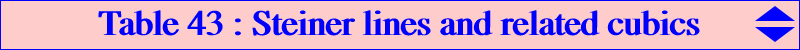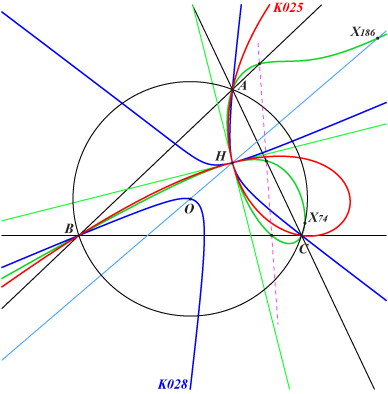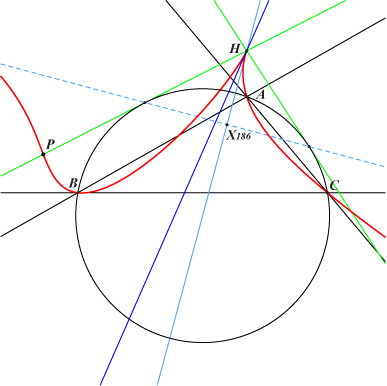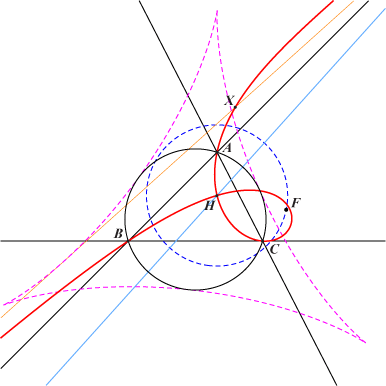Let M be a variable point on the circumcircle (O) of ABC and let (S) be the Steiner line of M, the line passing through the orthocenter H and parallel to the Simson line of M. Nodal cubics with node H Let P be a fixed point and let Q be the intersection of the lines (S) and PM. When M traverses (O), the locus of Q is a nodal circum-cubic (K) with node H passing through P. These cubics belong to a same net of cubics which can be generated by three decomposed cubics (Ka), (Kb), (Kc) where (Ka) is the union of the altitude AH and the rectangular circum-hyperbola tangent at A to (O) whose center is the midpoint of AH. Thus, we shall loosely write : (K) = p (Ka) + q (Kb) + r (Kc).Nodal tangents at H The nodal tangents at H are parallel to those of a circum-conic (C) passing through X(64) hence with perspector R on the trilinear polar of X(64).These tangents are therefore perpendicular if and only if (C) is the Jerabek hyperbola and then P must lie on the Euler line. This gives a pencil of cubics having all the same nodal tangents at H and meeting the Euler line again at a third point E. Each cubic of the pencil is then characterized by this point E. In particular, with E = G, H, O, X(30), X(376), X(3522) we obtain K1301, the union of the altitudes, K028 (a stelloid), K025 (a strophoid), K616, K917 respectively. When E is X(186), X(1113), X(1114) the cubic is a cK but the two latter cubics degenerate into one of the nodal tangents above and the rectangular hyperbola which is its X(393)-isoconjugate. The former cubic K1302 (in green on the figure) also contains X(74). Its root X(264) x X(523) is the trilinear pole of the line through X(125), X(136). With E = X(24), the cubic is the psK K620. See below.The cubic has a cusp at H if and only if (C) is a parabola therefore if and only if R lies on the Steiner inellipse and on the trilinear polar of X(64). These two curves have real common points when ABC is obtuse and, in this case, we obtain a cuspidal cubic. P must be a point lying on the two tangents drawn from H to the circumcircle. The cuspidal tangent is the Steiner line of the contact of the line PH with the circumcircle.Circular cubics (K) The cubic (K) is circular if and only if P lies on the line at infinity.In this case, • the singular focus F lies on C(H, R), • the real asymptote envelopes the deltoid with incircle (O), • the intersection X with the real asymptote lies on a complicated quintic. This circular cubic is in fact the antigonal of the line passing through H and P. It is a focal cubic when P = X(30) giving the strophoid K025 we met above. See figure opposite. It is an axial cubic when P is the infinite point of a sideline of the Morley triangle i.e. one of the infinite points of K024. Apart K025, the other remarkable examples are K186, K288, K289 obtained when P is X(523), X(1503), X(511) respectively.psK cubics (K) The cubic (K) meets the sidelines of ABC at U, V, W. If Ha, Hb, Hc are the reflections of H in the sidelines of ABC then U = BC /\ PHa, V = CA /\ PHb, W = AB /\ PHc. Note that the intersections U1, V1, W1 of PU, PV, PW with the Steiner lines of Ha, Hb, Hc respectively also lie on (K). The two triangles ABC and U1V1W1 are perspective at P1 on the cubic. Let us denote by tA, tB, tC its tangents at A, B, C. The following assertions are equivalent : UVW is the cevian triangle of a certain point Y, tA, tB, tC concur at a certain point Z, (K) is the cubic psK(Ω, Y, H). where Ω is the barycentric product Y x Z or H x Y/H. See here for psK cubics. In this case, the locus of P is K919, the isogonal transform of K044, passing through X(3), X(4), X(24), X(54), X(96), X(254), X(6193), X(8883), X(8884), the locus of Y is the pK with pole X393 x X276 and isopivot H, passing through X(4), X(264), X(275), X(317), X(2052), the locus of Z is the Thomson orthic cubic K350, passing through X(4), X(5), X(6), X(25), X(51), X(52), X(53), the locus of Ω is pK(X25 x X1093, X1093), passing through X(4), X(25), X(393), X(1093). With P = H, we obtain the union of the altitudes and with P = O, we obtain K028. The third possible point P on the Euler line is X(24) giving the cubic K620 with perpendicular nodal tangents and passing through X(4), X(24), X(64), X(1093), X(1118), X(2207).Asymptotes of (K) The asymptotes of the cubic (K) are parallel to those of pK(X6, P') where P' is the reflection of P in O. This confirms that (K) is a circular cubic if and only if P', and therefore P, lies on the line at infinity. This also shows that (K) is equilateral if and only if P = O giving K028 with three real asymptotes parallel to those of the McCay cubic K003. K616, obtained with P = X(376), has its asymptotes parallel to those of the Thomson cubic K002. Several other interesting examples are obtained with P = X(20), X(550) and X(40) giving the cubics K617, K618, K619 with asymptotes parallel to those of K006, K005 and to the bisectors of ABC respectively. *** (K) is a K+ with concurring asymptotes if and only if P lies on K664, a circum-cubic passing through O, H, X(83), X(550)*, the infinite points of pK(X6, X550), the points of K003 on (O). The isogonal transform of this cubic is a stelloid with radial center X(549). In this case, the point of concurrence of the asymptotes lies on another stelloid passing through X(4), X(5), X(381) and whose asymptotes are parallel to those of the McCay cubic K003.Points of (K) on the circumcircle The common points of (K) and the circumcircle (O) are six points also lying on the cubic pK(X6, Z) where Z is the anticomplement of the X(3)-isoconjugate of the Ceva point of X(6) and P. With P = p : q : r, the first coordinate of Z is : a^2 [b^2 c^2 p + c^2 (a^2 - c^2) q + b^2 (a^2 - b^2) r]. The mapping f : P -> Z is a homography with fixed points X(110), X(2574), X(2575) which also globally fixes the line at infinity. In particular, f(X3) = X4.Miscellaneous other properties of (K) • (K) is a K0 (without term in xyz) if and only if P lies on the line HK. • The points U, V, W above are collinear if and only if P lies on (O) – which gives decomposed nK cubics – or on the line X(186)X(523). With P = X186 we find cK(#X4, X264 x X523) already mentioned and with P = X(523) we find K186. • The orthic line (D) of (K) passes through X(5) and cP, the complement of P. This line is undefined when P = O since the corresponding cubic (K) is the stelloid K028. If P ≠ O, this means that the polar conic of any point on (D) is a rectangular hyperbola and, in particular, the polar conic of X(5) in every cubic (K) is a rectangular hyperbola. Note that (K) contains X(5) when P lies on the line passing through X(5), X(49), X(54), X(110), X(265), X(567), X(1141), etc, giving a pencil of cubics (K) generated by the two decomposed cubics obtained with P = X(110), X(1141). • More generally, (K) contains a given point M ≠ H, M not on (O), if and only if P lies on the line passing through M and the fourth intersection E of (O) with the rectangular circum-hyperbola passing through M. The line ME meets (O) again at E'. All the cubics (K) with P on ME belong to a same pencil generated by the two decomposed cubics obtained with P = E, P = E'. In both cases, (K) is the union of the Steiner line of P and the rectangular circum-hyperbola passing through P. It follows that all these cubics must contain a nineth common point which is the intersection M' of ME and the Steiner line of E. Remark 1 : E = E' if and only if M = M' if and only if M lies on the cubic K027 = cK(#X4, X2052) = nK(X393, X2052, X4) passing through X(i) for i = 4, 10412, 14380, 15329, 43665, 43728. Remark 2 : when M lies on K621 = pK(X393, X2052), there is one point P for which the cubic (K) contains the vertices of the anticevian triangle of M. This is in particular the case when M is one of the points X(2) giving K617, X(4), X(6) giving K622, X(24), X(393), X(847), X(2052).The following table gathers all mentioned cubics together with several other interesting examples. ∞Knnn means that the cubic contains the infinite points of Knnn. Knnn* is the isogonal transform of Knnn.P cubic Xi on the cubic for i = type remarks X(2) K1301 2, 4, 3434, 4846, 11185, 14593, 30513, 34029, 41370, 52448, 52449, 52450, 52451, 52452, 52453, 52454, 52455, 52456, ∞K243 anticomplement of K1265 X(3) K028 3, 4, 8, 76, 847, 3557, 3558, 3730, 8743, 10571, ∞K003 psK a stelloid, K009* X(4) 4, ∞K004 K0+ union of the altitudes X(6) K622 3, 4, 1614 K0 X(20) K617 4, 20, 68, 254, 315, 2996, 3436, ∞K006 anticomplement of K009, K389* X(24) K620 4, 24, 64, 1093, 1118, 2207 psK X(30) K025 4, 30, 265, 316, 671, 1263, 1300, 5080, 5134, 5203, 5523, 5962, 10152, 11604, 11605, ∞K001 a strophoid, K039* X(40) K619 4, 40, 3680, 3869, ∞bisectors X(54) 4, 5, 54, 217, ∞pK(X6, X7691) psK X(83) 4, 83 K+ X(186) K1302 4, 74, 186, 6344, 8744, 8753, 44146, 46105 cK X(376) K616 4, 69, 376, 1249, 3421, 5485, 6601, 9214, ∞K002 K626* X(511) K289 4, 23, 511, 895, 1337, 1338, 1916, 3557, 3558, 3563, 11609, 11610 circular, K1281* X(523) K186 4, 107, 523, 7471 cK circular X(550) K618 4, 550, 3471, 3519, 7768, ∞K005 X(1350) 4, 22, 1350 circular X(1503) K288 4, 67, 98, 858, 1503 K0 circular X(3522) K917 4, 3522,∞K832Remark : with P on the Euler line (yellow cells), the cubics are in a same pencil and the nodal tangents at H are parallel to the asymptotes of the Jerabek hyperbola. The points at infinity are those of a cubic of the Euler pencil.